# 收藏 | 10个重要问题概览Transformer全部内容

AI博士笔记系列推荐

Github 上有与文章配套的 jupyter notebook，和文章配合食用，效果更佳。

https://github.com/BSlience/transformer-all-in-one

• Attention 机制是用来做什么的 ？

• Self-attention 是怎么从 Attention 过度过来的 ？

• Attention 和 self-attention 的区别是什么 ？

• Self-attention 为什么能 work ？

• 怎么用 Pytorch 实现 self-attention ？

• Transformer 的作者对 self-attention 做了哪些 tricks ？

• 怎么用 Pytorch/Tensorflow2.0 实现在 Transfomer 中的 self-attention ？

• 完整的 Transformer Block 是什么样的？

• 怎么捕获序列中的顺序信息呢 ？

• 怎么用 Pytorch 实现一个完整的 Transformer 模型？

## Attention 机制是用来做什么的 ？

Attention机制最早的提出是针对与序列模型的，出处是Bengio大神在2015年的这篇文章：

Neural Machine Translation by jointly learning to align and translate, Bengio et. al. ICLR 2015

https://arxiv.org/pdf/1409.0473.pdf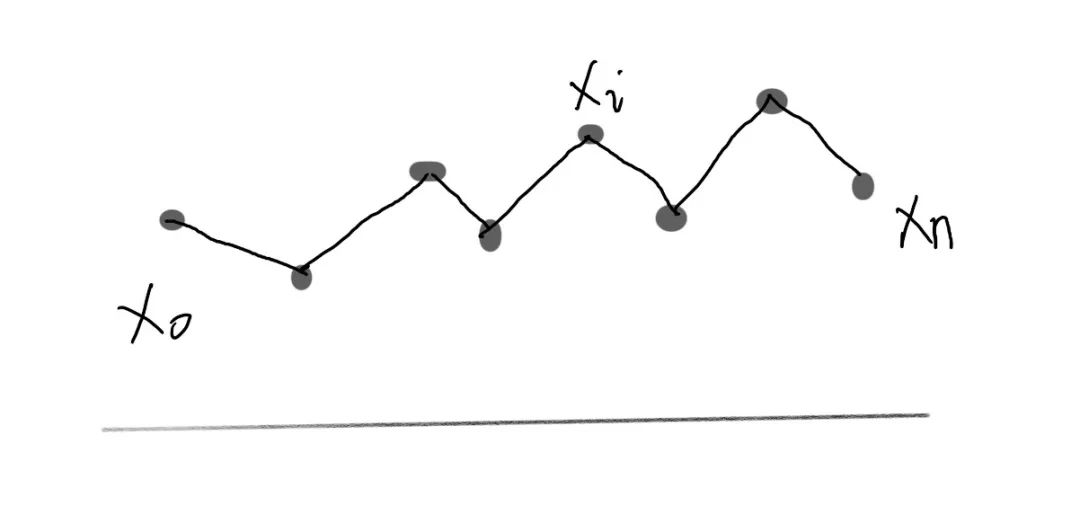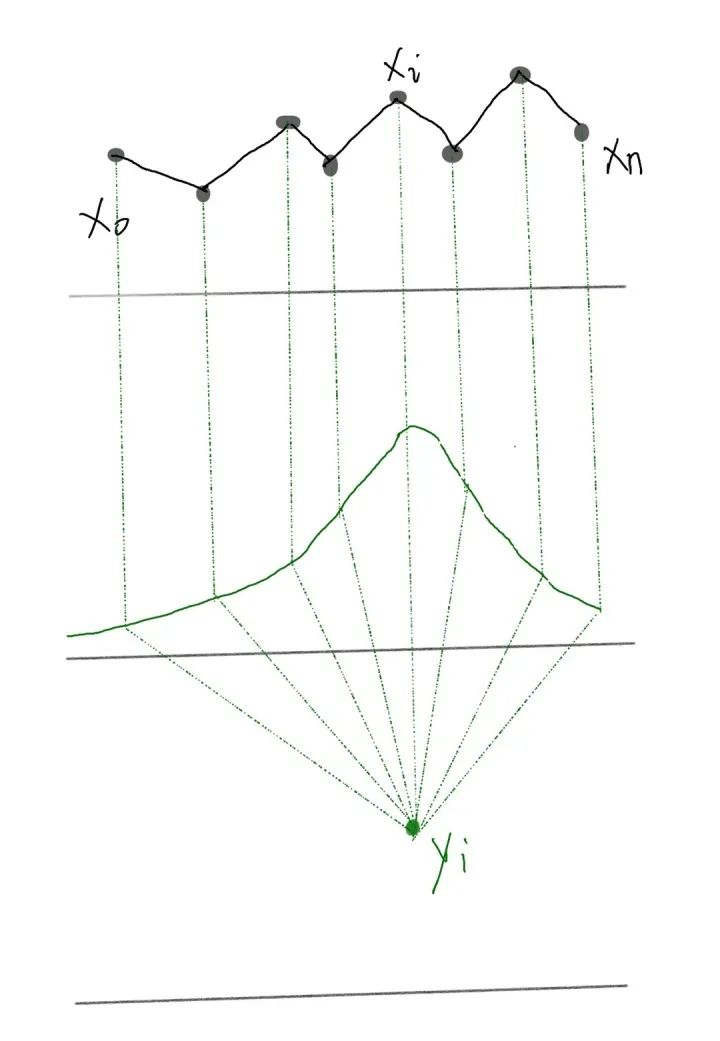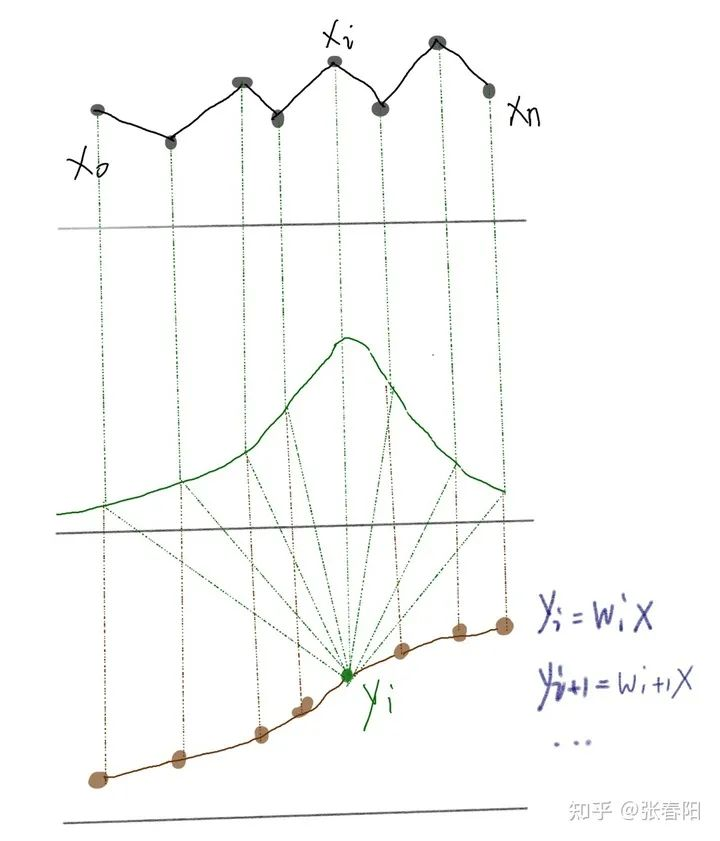Self-attention是一个序列到序列的操作：一个向量的序列作为输入，一个向量的序列作为输出。我们把输入的序列定义为   ，并且与它相关的输出向量是   。这两个向量的维度都是   。那么对于每一个点   ，我们都可以通过一个不同的权重值，来将它转化为一个新的序列，这个新的序列就可能是我们原始序列的一个更好的表示，这些   就是一组attention的值。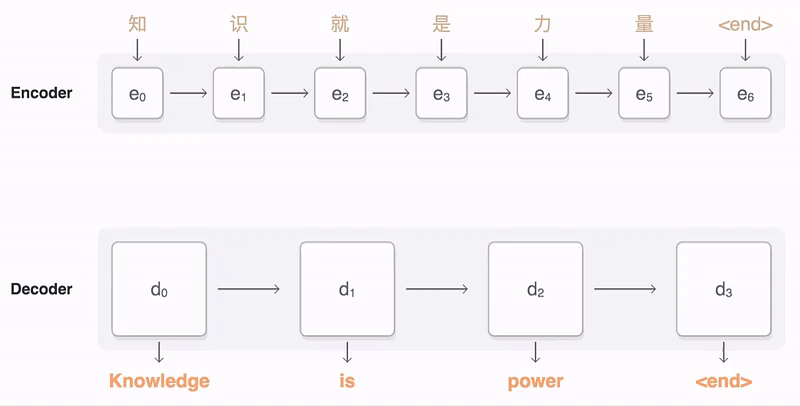## Self-attention 是怎么从 Attention 过度过来的 ？

Self-attention就本质上是一种特殊的attention。它和attention的区别我会在下一个章节介绍，这里先来介绍下self-attention，这种应用在transformer中最重要的结构之一。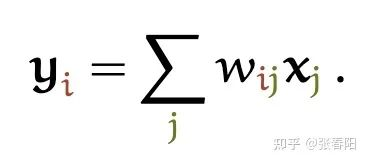代表整个序列的长度，并且   个权重的相加之和等于1。值得一提的是，这里的   并不是一个需要神经网络学习的参数，它是来源于   和   的之间的计算的结果（这里   的计算发生了变化）。它们之间最简单的一种计算方式，就是使用点积的方式。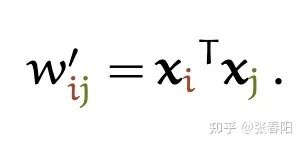和   是一对输入和输出。对于下一个输出的向量   ，我们有一个全新的输入序列和一个不同的权重值。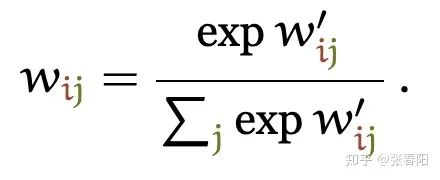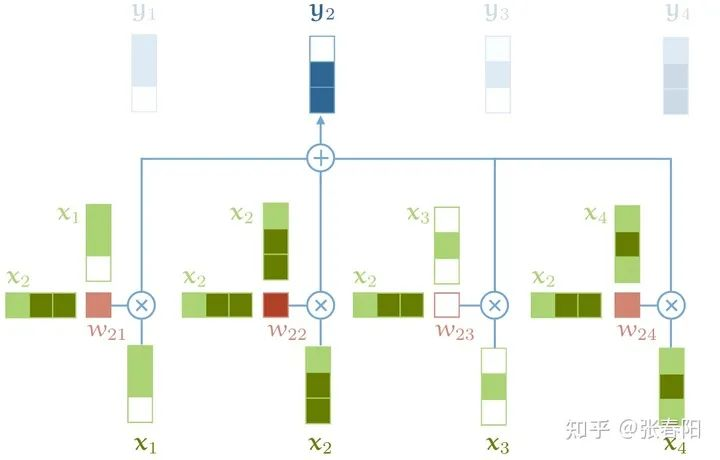self-attention的基础操作，没有包含softmax操作

## Attention 和 self-attention 的区别是什么 ？

1. 在神经网络中，通常来说你会有输入层（input），应用激活函数后的输出层（output），在RNN当中你会有状态（state）。如果attention (AT) 被应用在某一层的话，它更多的是被应用在输出或者是状态层上，而当我们使用self-attention（SA），这种注意力的机制更多的实在关注input上。

2. Attention (AT) 经常被应用在从编码器（encoder）转换到解码器（decoder）。比如说，解码器的神经元会接受一些AT从编码层生成的输入信息。在这种情况下，AT连接的是两个不同的组件（component），编码器和解码器。但是如果我们用SA，它就不是关注的两个组件，它只是在关注你应用的那一个组件。那这里他就不会去关注解码器了，就比如说在Bert中，使用的情况，我们就没有解码器。

3. SA可以在一个模型当中被多次的、独立的使用（比如说在Transformer中，使用了18次；在Bert当中使用12次）。但是，AT在一个模型当中经常只是被使用一次，并且起到连接两个组件的作用。

4. SA比较擅长在一个序列当中，寻找不同部分之间的关系。比如说，在词法分析的过程中，能够帮助去理解不同词之间的关系。AT却更擅长寻找两个序列之间的关系，比如说在翻译任务当中，原始的文本和翻译后的文本。这里也要注意，在翻译任务重，SA也很擅长，比如说Transformer。

5. AT可以连接两种不同的模态，比如说图片和文字。SA更多的是被应用在同一种模态上，但是如果一定要使用SA来做的话，也可以将不同的模态组合成一个序列，再使用SA。

6. 对我来说，大部分情况，SA这种结构更加的general，在很多任务作为降维、特征表示、特征交叉等功能尝试着应用，很多时候效果都不错。

## Self-attention 为什么能 work ？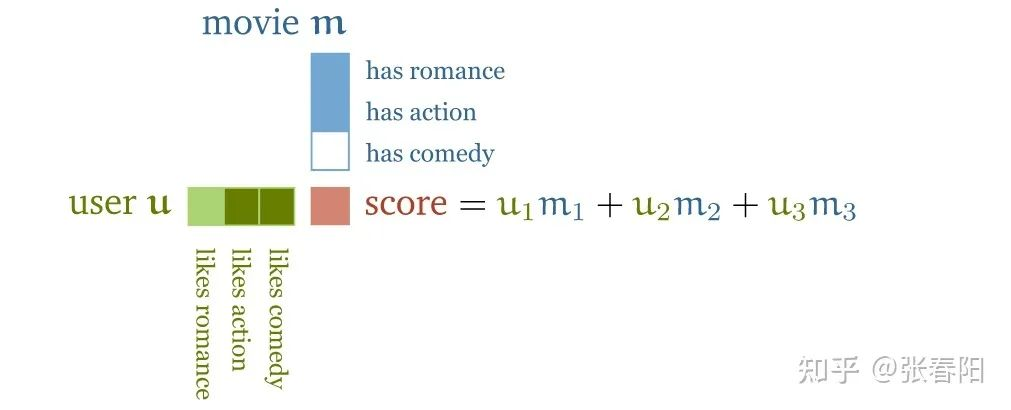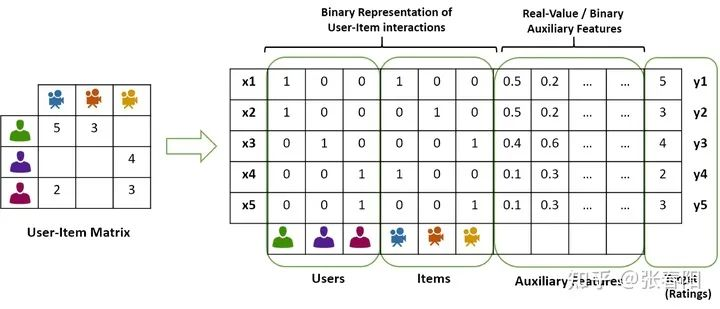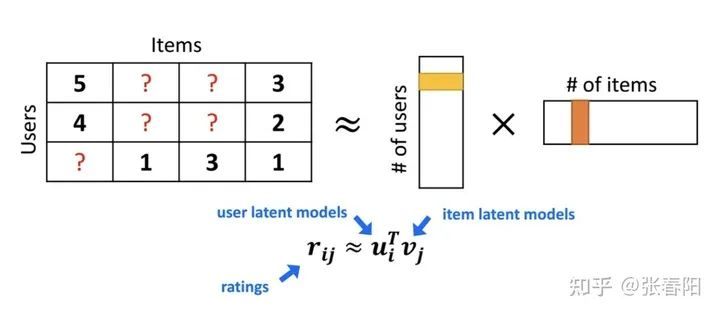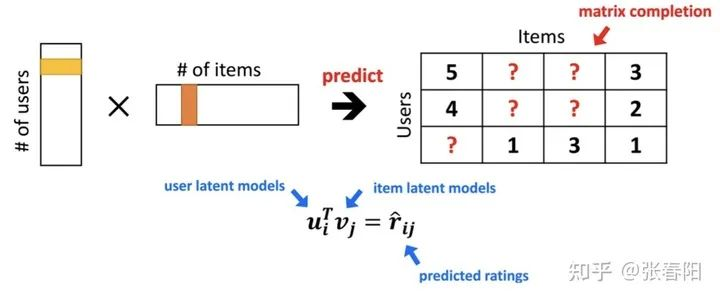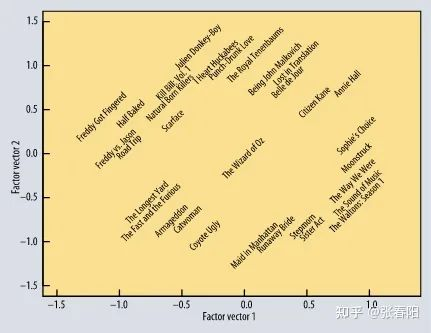• 到目前为止，我们还没有用到需要学习的参数。基础的self-attention实际上完全取决于我们创建的输入序列，上游的embeding layer驱动着self-attention学习对于文本语义的向量表示。

• Self-attention看到的序列只是一个集合(set)，不是一个序列，它并没有顺序。如果我们重新排列集合，输出的序列也是一样的。后面我们要使用一些方法来缓和这种没有顺序所带来的信息的缺失。但是值得一提的是，self-attention本身是忽略序列的自然输入顺序的。

## 怎么用 Pytorch 实现self-attention ？

-- 费曼

import torchimport torch.nn.functional as F
# assume we have some tensor x with size (b, t, k)x = ...
raw_weights = torch.bmm(x, x.transpose(1, 2))# - torch.bmm is a batched matrix multiplication. It #   applies matrix multiplication over batches of #   matrices.



weights = F.softmax(raw_weights, dim=2)


y = torch.bmm(weights, x)

# Transformer 的作者对 Self-attention 做了哪些 tricks ？

1) Queries, keys and values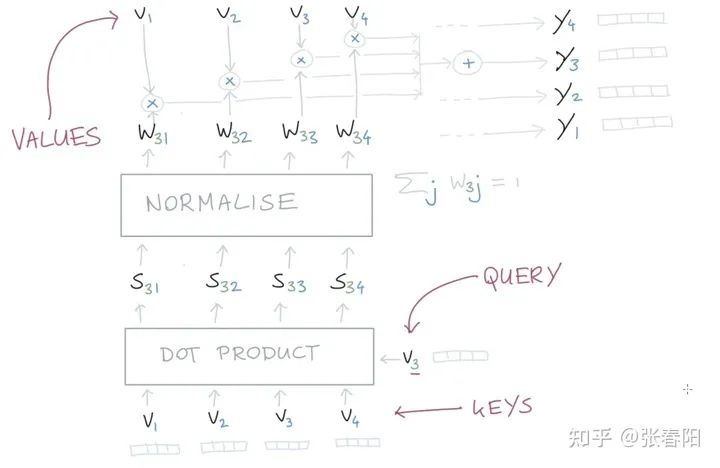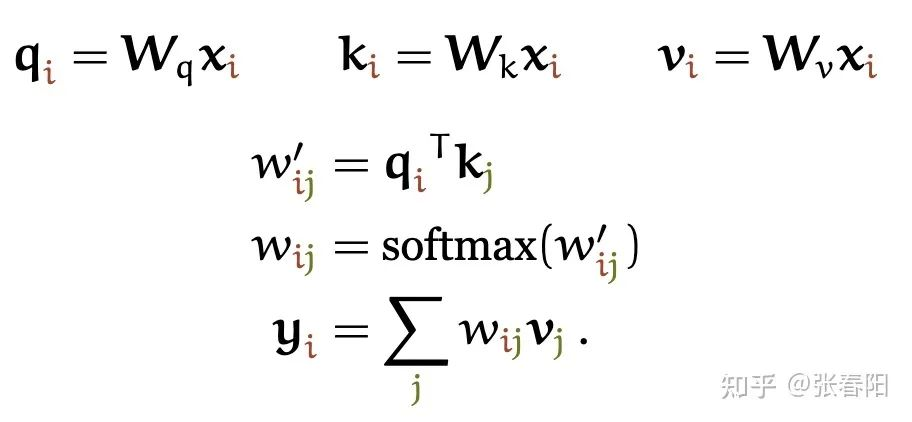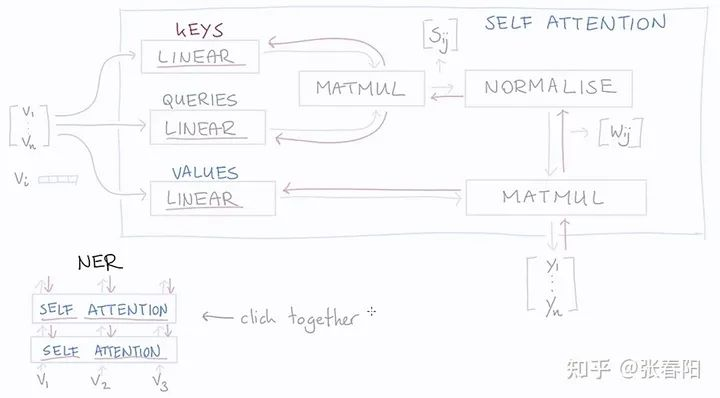Linear层是一个没有bias的全连接层，其实就是一个点乘。红色的箭头表示的是反向传播的过程。通过方向传播，key，query，value就能够学习到一个合理的表示。那么这里面key，queue，value分别学习到的是什么呢？这个可能并没有一个官方的解释，但是通过这三个名称的命名方式，我们可以大致的猜测。

2) 缩放点积的值(Scaling the dot product)

Softmax 函数对非常大的输入很敏感。这会使得梯度的传播出现为问题(kill the gradient)，并且会导致学习的速度下降(slow down learning)，甚至会导致学习的停止。那如果我们使用   来对输入的向量做缩放，就能够防止进入到softmax的函数增长过大：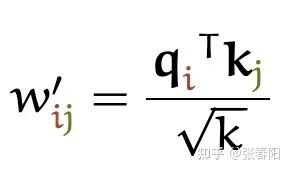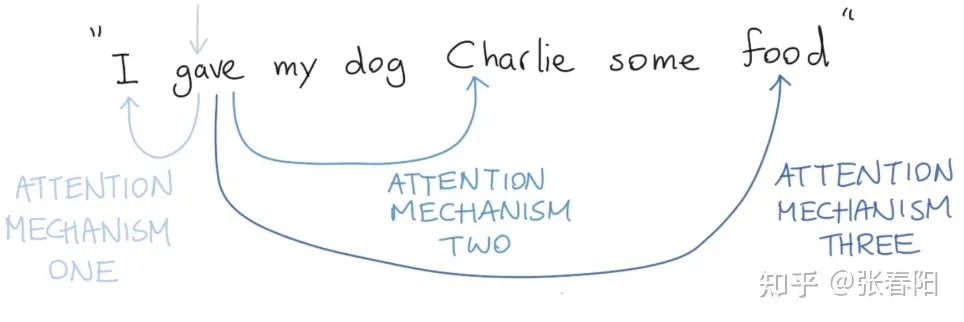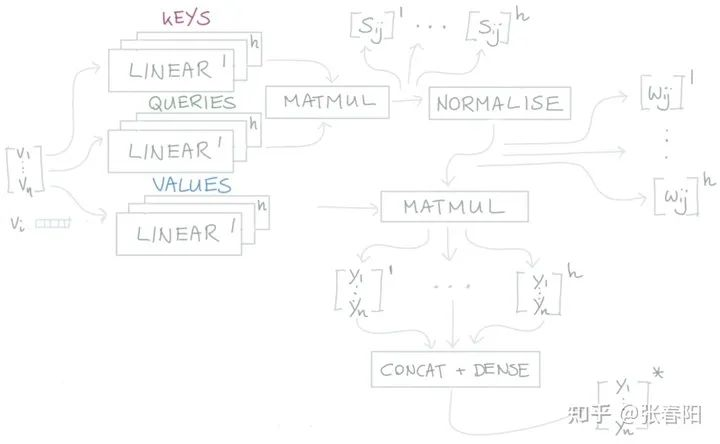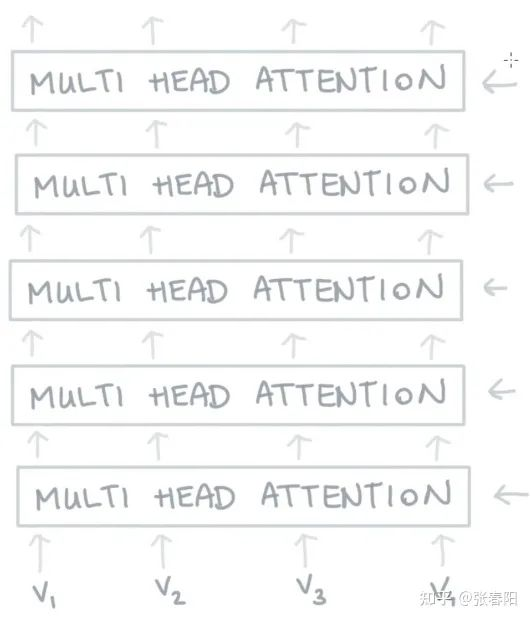Narrow and wide self-attention

## 怎么用 Pytorch/Tensorflow2.0 实现在 Transfomer 中的self-attention ？

1. 准备输入

2. 初始化参数

3. 获取key，query和value

4. 给input1计算attention score

5. 计算softmax

6. 给value乘上score

7. 给value加权求和获取output1

8. 重复步骤4-7，获取output2，output3

1 准备输入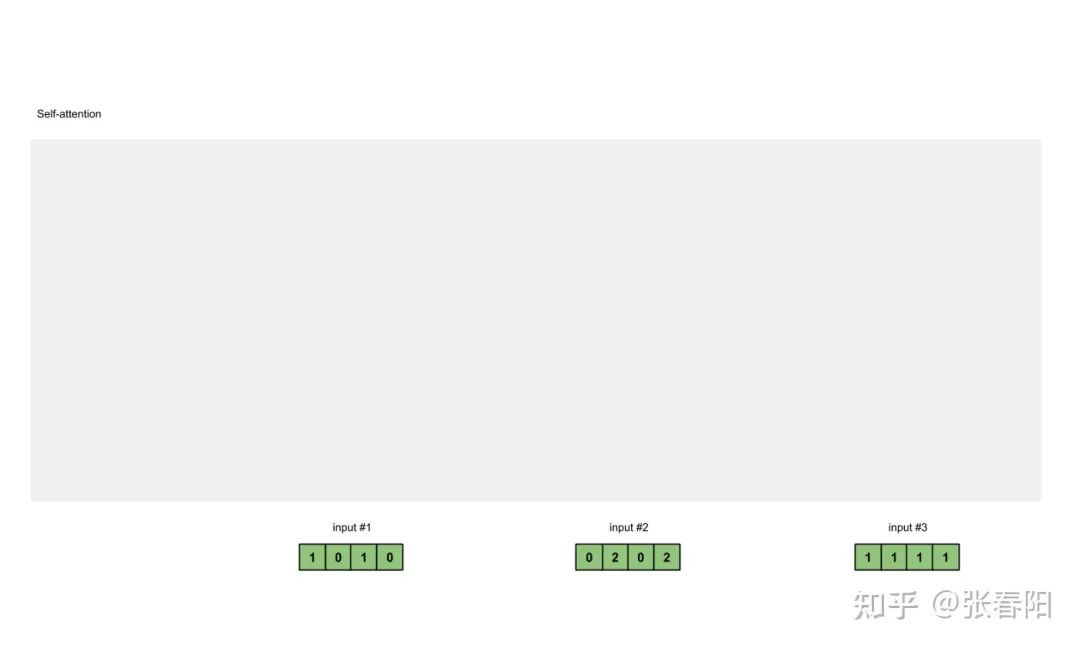Input 1: [1, 0, 1, 0]
Input 2: [0, 2, 0, 2]
Input 3: [1, 1, 1, 1]

2 初始化参数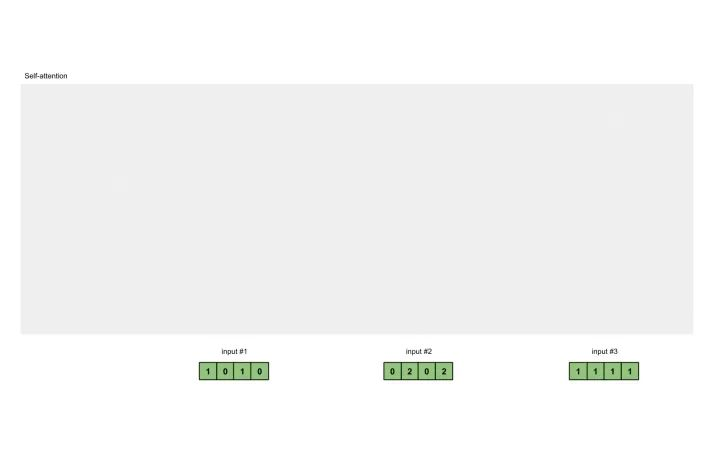key的参数：

[[0, 0, 1], [1, 1, 0], [0, 1, 0], [1, 1, 0]]
query的参数：

[[1, 0, 1], [1, 0, 0], [0, 0, 1], [0, 1, 1]]
value的参数：

[[0, 2, 0],
[0, 3, 0],
[1, 0, 3],
[1, 1, 0]]

通常在神经网络的初始化过程中，这些参数都是比较小的，一般会在_Gaussian,
Xavier and Kaiming distributions随机采样完成。_


3 获取key，query和value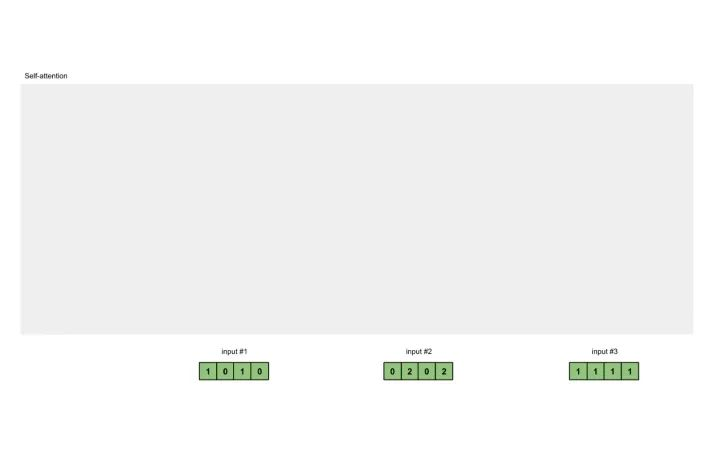[0, 0, 1][1, 0, 1, 0] x [1, 1, 0] = [0, 1, 1]               [0, 1, 0]               [1, 1, 0]



               [0, 0, 1][0, 2, 0, 2] x [1, 1, 0] = [4, 4, 0]               [0, 1, 0]               [1, 1, 0]



               [0, 0, 1][1, 1, 1, 1] x [1, 1, 0] = [2, 3, 1]               [0, 1, 0]               [1, 1, 0]



               [0, 0, 1][1, 0, 1, 0]   [1, 1, 0]   [0, 1, 1][0, 2, 0, 2] x [0, 1, 0] = [4, 4, 0][1, 1, 1, 1]   [1, 1, 0]   [2, 3, 1]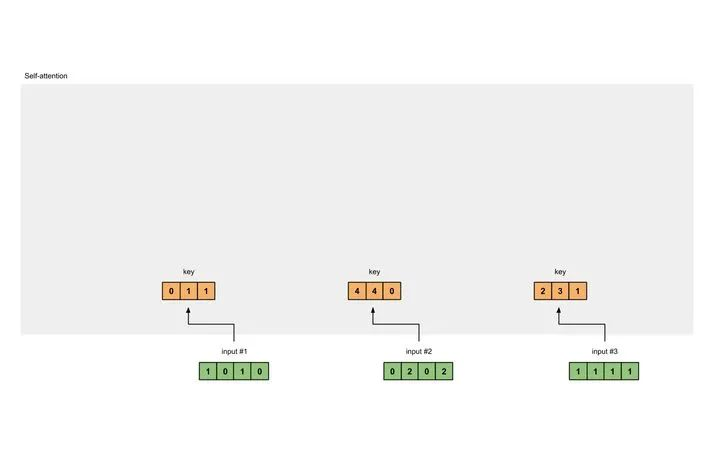[0, 2, 0][1, 0, 1, 0]   [0, 3, 0]   [1, 2, 3] [0, 2, 0, 2] x [1, 0, 3] = [2, 8, 0][1, 1, 1, 1]   [1, 1, 0]   [2, 6, 3]



query也是一样的。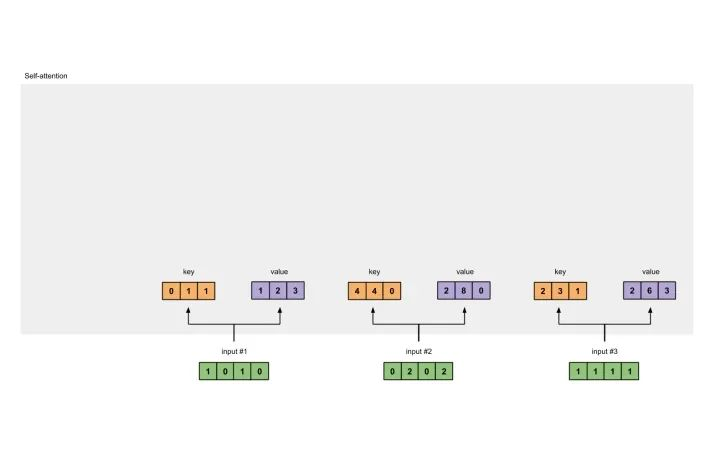[1, 0, 1][1, 0, 1, 0]   [1, 0, 0]   [1, 0, 2][0, 2, 0, 2] x [0, 0, 1] = [2, 2, 2][1, 1, 1, 1]   [0, 1, 1]   [2, 1, 3]



4 给input1计算attention score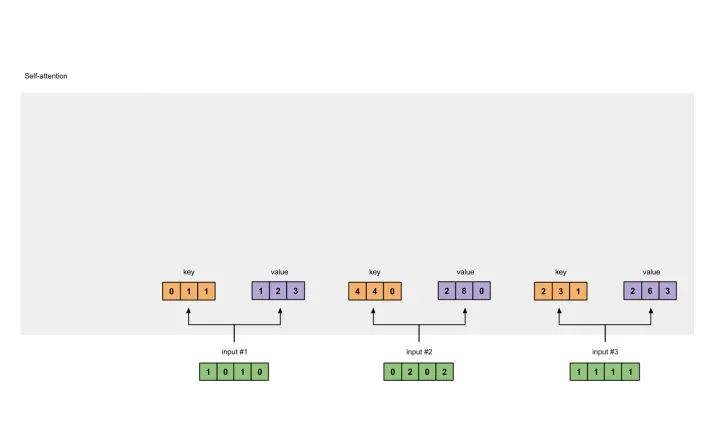[0, 4, 2]
[1, 0, 2] x [1, 4, 3] = [2, 4, 4]
[1, 0, 1]


5 计算softmax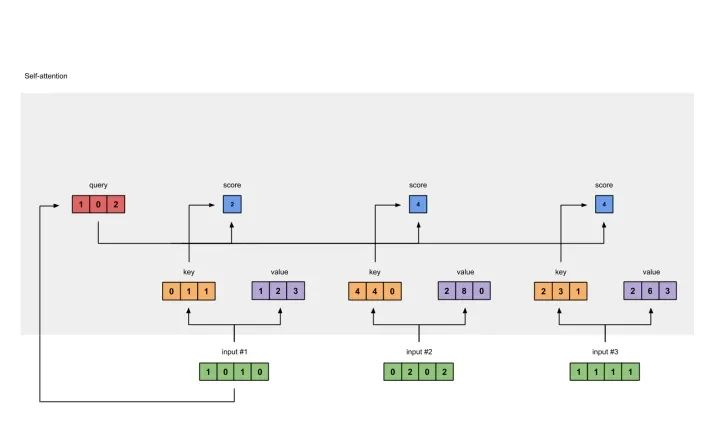softmax([2, 4, 4]) = [0.0, 0.5, 0.5]



6 给value乘上score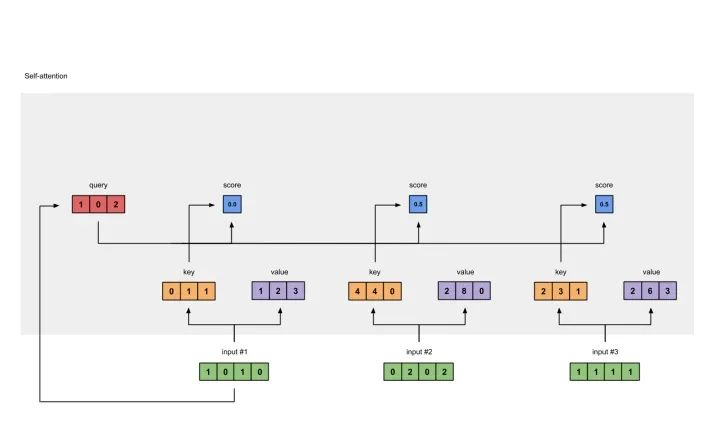1: 0.0 * [1, 2, 3] = [0.0, 0.0, 0.0]2: 0.5 * [2, 8, 0] = [1.0, 4.0, 0.0]3: 0.5 * [2, 6, 3] = [1.0, 3.0, 1.5]



7 给value加权求和获取output1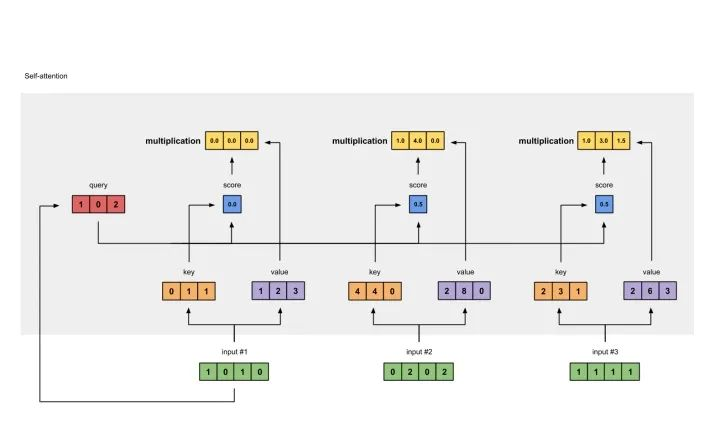[0.0, 0.0, 0.0]+ [1.0, 4.0, 0.0]+ [1.0, 3.0, 1.5]-----------------= [2.0, 7.0, 1.5]



8 重复步骤4-7，获取output2，output3https://github.com/BSlience/transformer-all-in-onegithub.com

# 完整的 Transformer Block 是什么样的？

Transformer 模型来源于Google发表的一篇论文 “Attention Is All You Need”，截止到我查询的时候，这篇文章已经有17000+的引用量，可见这篇文章的影响力。希望大家能在有一些了解的基础上，能够自己读一下这篇文章。

https://arxiv.org/pdf/1706.03762.pdfarxiv.org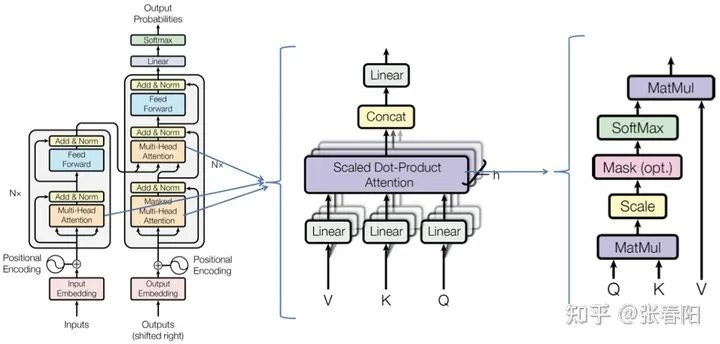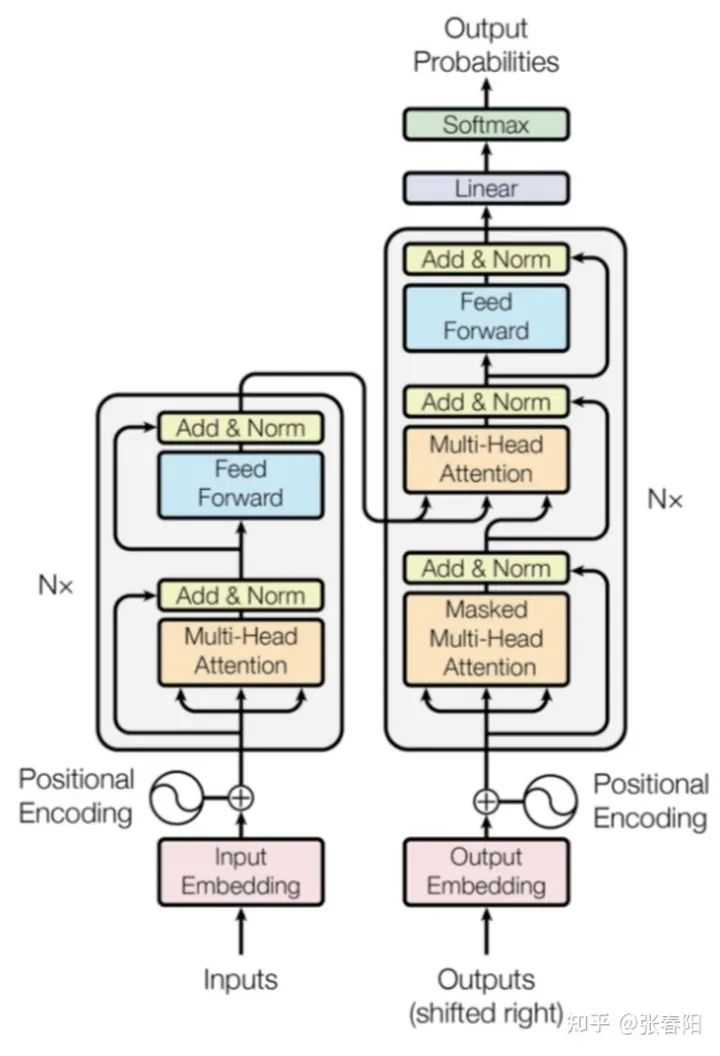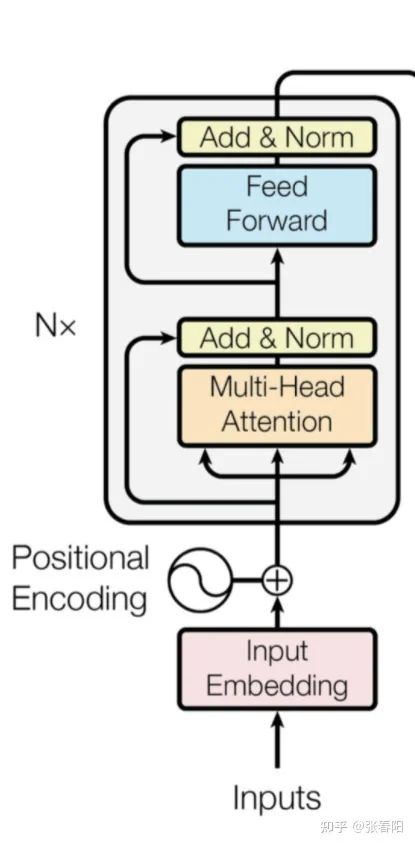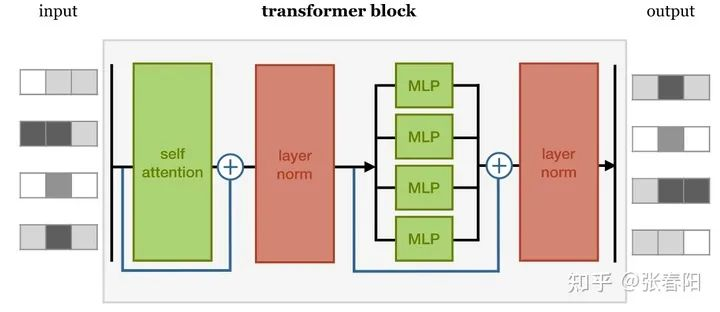1. self-attention layer

2. normalization layer

3. feed forward layer

4. another normalization layer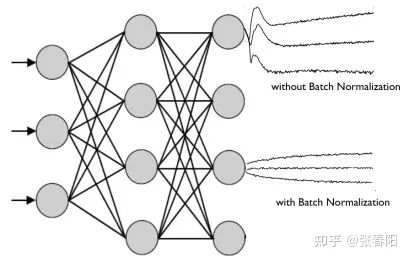Normaliztion 和 residual connections 是我们经常使用的，帮助加快深度神经网络训练速度和准确率的 tricks。

class TransformerBlock(nn.Module):  def __init__(self, k, heads):    super().__init__()
self.norm1 = nn.LayerNorm(k)    self.norm2 = nn.LayerNorm(k)
self.ff = nn.Sequential(      nn.Linear(k, 4 * k),      nn.ReLU(),      nn.Linear(4 * k, k))
def forward(self, x):    attended = self.attention(x)    x = self.norm1(attended + x)        fedforward = self.ff(x)    return self.norm2(fedforward + x)



## 怎么捕获序列中的顺序信息呢 ？

• position embeddings

• position encodings

position encoding的方法其实和 position embedding 的方法很相似，我们都是希望能够通过一个位置的 vector 来表示位置的信息，让模型学习到这个信息。但是，这里稍有不同的是，encoding 的方法是由我们选择一个 function 来生成每个位置的 vector 的，并且让模型网络去找出该如何去理解这些 encoding vector。这样做的好处是，对于一个选择的比较好的function，网络模型能够处理那些在训练阶段没有见过的序列位置 vector（虽然这也并不是说这些没见过的位置 vector 一定能够表现的很好，但是好在是我们可以有比较直接的方法来测试他们）。这种方法也是 Transformer 的作者选择的方法，让我们看看作者是怎么设计这个 function 的。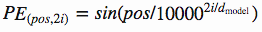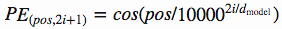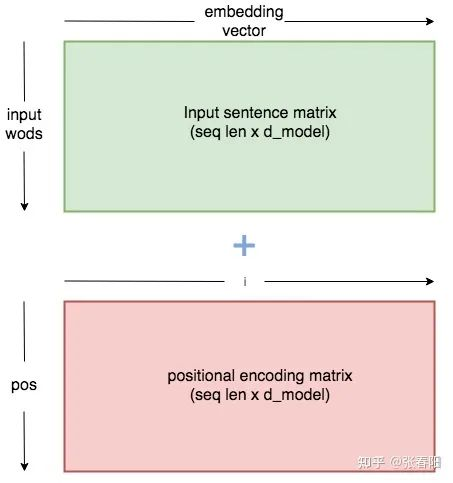class PositionalEncoder(nn.Module):    def __init__(self, d_model, max_seq_len = 80):        super().__init__()        self.d_model = d_model                # 根据pos和i创建一个常量pe矩阵        pe = torch.zeros(max_seq_len, d_model)        for pos in range(max_seq_len):            for i in range(0, d_model, 2):                pe[pos, i] = \                math.sin(pos / (10000 ** ((2 * i)/d_model)))                pe[pos, i + 1] = \                math.cos(pos / (10000 ** ((2 * (i + 1))/d_model)))                        pe = pe.unsqueeze(0)        self.register_buffer('pe', pe)     def forward(self, x):        # 让 embeddings vector 相对大一些        x = x * math.sqrt(self.d_model)        # 增加位置常量到 embedding 中        seq_len = x.size(1)        x = x + Variable(self.pe[:,:seq_len], \                         requires_grad=False).cuda()        return x



## 怎么用 Pytorch 实现一个完整的 Transformer 模型？

1. Tokenize

2. Input Embedding

3. Positional Encoder

4. Transformer Block

5. Encoder

6. Decoder

7. Transformer

1 Tokenize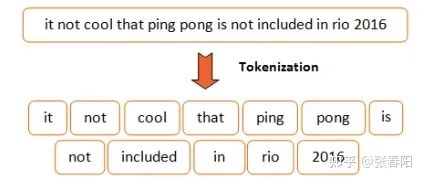class Tokenize(object):

def __init__(self, lang):

def tokenizer(self, sentence):
sentence = re.sub(
r"[\*\"“”\n\\…\+\-\/\=‘•:\|’\!;]", " ", str(sentence))
sentence = re.sub(r"[ ]+", " ", sentence)
sentence = re.sub(r"\!+", "!", sentence)
sentence = re.sub(r"\,+", ",", sentence)
sentence = re.sub(r"\?+", "?", sentence)
sentence = sentence.lower()
return [tok.text for tok in self.nlp.tokenizer(sentence) if tok.text != " "]


2 Input Embedding

Token Embedding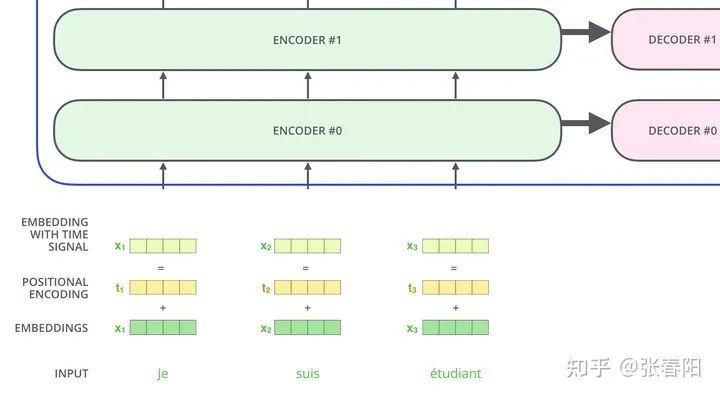class Embedding(nn.Module):        def __init__(self, vocab_size, d_model):        super().__init__()        self.d_model = d_model        self.embed = nn.Embedding(vocab_size, d_model)            def forward(self, x):        return self.embed(x)



Positional Encoderclass PositionalEncoder(nn.Module):

def __init__(self, d_model, max_seq_len = 80):
super().__init__()
self.d_model = d_model

# 根据pos和i创建一个常量pe矩阵
pe = torch.zeros(max_seq_len, d_model)
for pos in range(max_seq_len):
for i in range(0, d_model, 2):
pe[pos, i] = \
math.sin(pos / (10000 ** ((2 * i)/d_model)))
pe[pos, i + 1] = \
math.cos(pos / (10000 ** ((2 * (i + 1))/d_model)))

pe = pe.unsqueeze(0)
self.register_buffer('pe', pe)

def forward(self, x):
# 让 embeddings vector 相对大一些
x = x * math.sqrt(self.d_model)
# 增加位置常量到 embedding 中
seq_len = x.size(1)
x = x + Variable(self.pe[:,:seq_len], \
return x

3 Transformer Block


1. self-attention layer

2. normalization layer

3. feed forward layer

4. another normalization layerAttention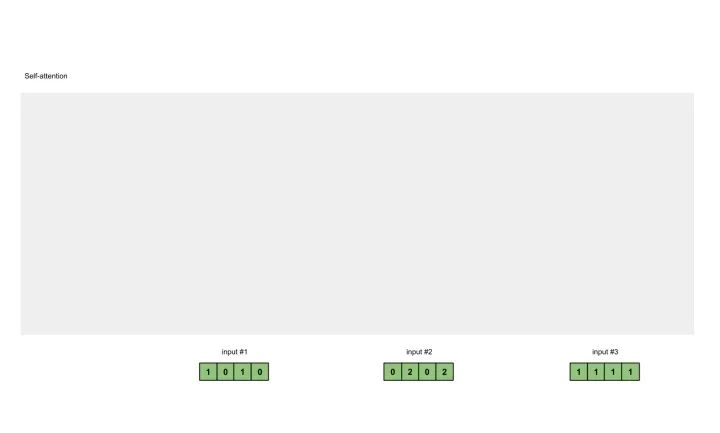def attention(q, k, v, d_k, mask=None, dropout=None):        scores = torch.matmul(q, k.transpose(-2, -1)) /  math.sqrt(d_k)        # mask掉那些为了padding长度增加的token，让其通过softmax计算后为0    if mask is not None:        mask = mask.unsqueeze(1)        scores = scores.masked_fill(mask == 0, -1e9)        scores = F.softmax(scores, dim=-1)        if dropout is not None:        scores = dropout(scores)            output = torch.matmul(scores, v)    return output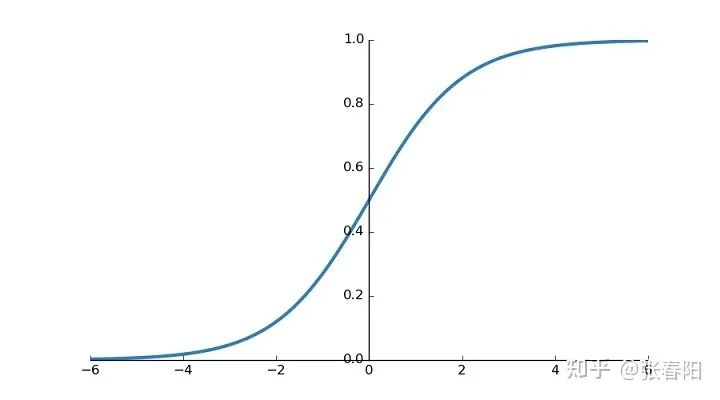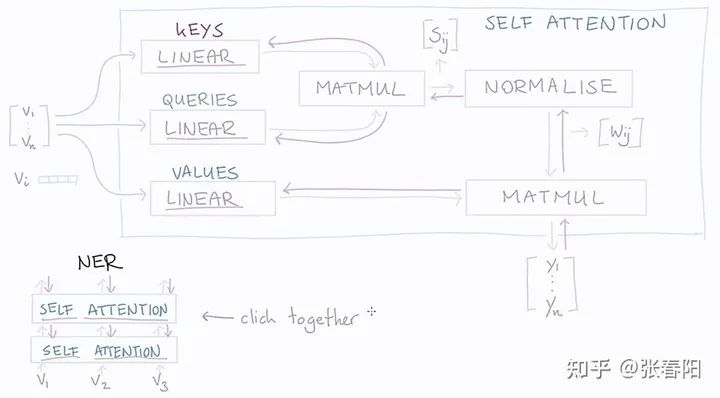class MultiHeadAttention(nn.Module):        def __init__(self, heads, d_model, dropout = 0.1):        super().__init__()                self.d_model = d_model        self.d_k = d_model // heads        self.h = heads                self.q_linear = nn.Linear(d_model, d_model)        self.v_linear = nn.Linear(d_model, d_model)        self.k_linear = nn.Linear(d_model, d_model)                self.dropout = nn.Dropout(dropout)        self.out = nn.Linear(d_model, d_model)        def forward(self, q, k, v, mask=None):                bs = q.size(0)                # perform linear operation and split into N heads        k = self.k_linear(k).view(bs, -1, self.h, self.d_k)        q = self.q_linear(q).view(bs, -1, self.h, self.d_k)        v = self.v_linear(v).view(bs, -1, self.h, self.d_k)                # transpose to get dimensions bs * N * sl * d_model        k = k.transpose(1,2)        q = q.transpose(1,2)        v = v.transpose(1,2)                # calculate attention using function we will define next        scores = attention(q, k, v, self.d_k, mask, self.dropout)        # concatenate heads and put through final linear layer        concat = scores.transpose(1,2).contiguous()\        .view(bs, -1, self.d_model)        output = self.out(concat)            return output



Layer Norm

https://arxiv.org/pdf/2003.07845.pdfarxiv.org

class NormLayer(nn.Module):        def __init__(self, d_model, eps = 1e-6):        super().__init__()            self.size = d_model                # 使用两个可以学习的参数来进行 normalisation        self.alpha = nn.Parameter(torch.ones(self.size))        self.bias = nn.Parameter(torch.zeros(self.size))                self.eps = eps        def forward(self, x):        norm = self.alpha * (x - x.mean(dim=-1, keepdim=True)) \        / (x.std(dim=-1, keepdim=True) + self.eps) + self.bias        return norm



Feed Forward Layer

class FeedForward(nn.Module):        def __init__(self, d_model, d_ff=2048, dropout = 0.1):        super().__init__()             # We set d_ff as a default to 2048        self.linear_1 = nn.Linear(d_model, d_ff)        self.dropout = nn.Dropout(dropout)        self.linear_2 = nn.Linear(d_ff, d_model)        def forward(self, x):        x = self.dropout(F.relu(self.linear_1(x)))        x = self.linear_2(x)



5 Encoder

Encoder 就是将上面讲解的内容，按照下图堆叠起来，完成将源编码到中间编码的转换。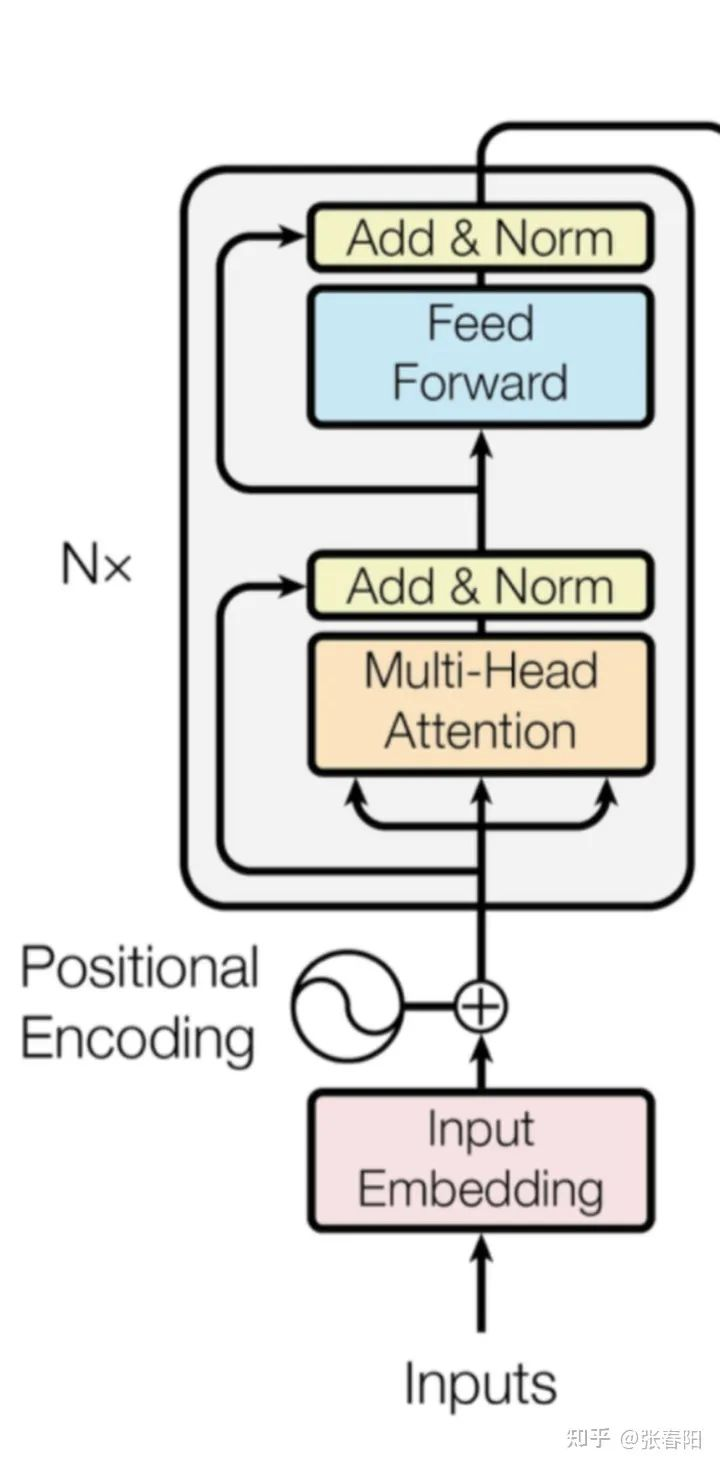class EncoderLayer(nn.Module):
def __init__(self, d_model, heads, dropout=0.1):        super().__init__()        self.norm_1 = Norm(d_model)        self.norm_2 = Norm(d_model)        self.attn = MultiHeadAttention(heads, d_model, dropout=dropout)        self.ff = FeedForward(d_model, dropout=dropout)        self.dropout_1 = nn.Dropout(dropout)        self.dropout_2 = nn.Dropout(dropout)            def forward(self, x, mask):        x2 = self.norm_1(x)        x = x + self.dropout_1(self.attn(x2,x2,x2,mask))        x2 = self.norm_2(x)        x = x + self.dropout_2(self.ff(x2))        return x

class Encoder(nn.Module):
def __init__(self, vocab_size, d_model, N, heads, dropout):        super().__init__()        self.N = N        self.embed = Embedder(vocab_size, d_model)        self.pe = PositionalEncoder(d_model, dropout=dropout)        self.layers = get_clones(EncoderLayer(d_model, heads, dropout), N)        self.norm = Norm(d_model)
def forward(self, src, mask):        x = self.embed(src)        x = self.pe(x)        for i in range(self.N):            x = self.layers[i](x, mask)        return self.norm(x)



6 Decoder

Decoder部分和 Encoder 的部分非常的相似，它主要是把 Encoder 生成的中间编码，转换为目标编码。后面我会在具体的任务中，来分析它和 Encoder 的不同。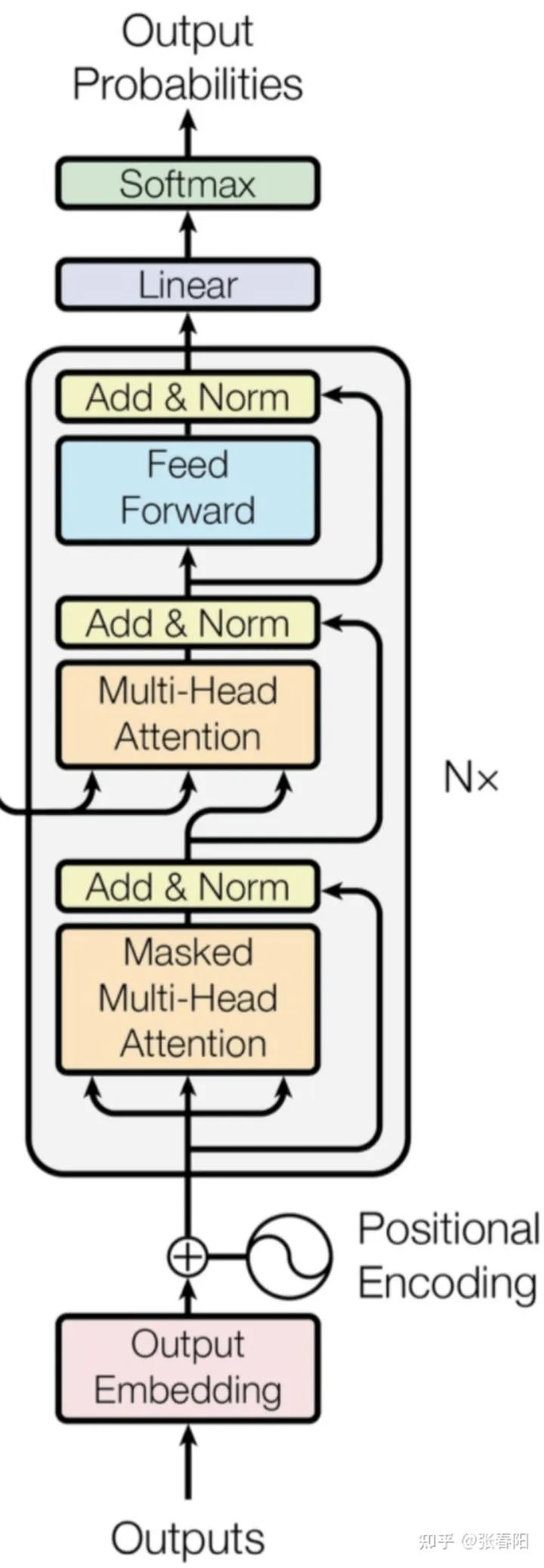class DecoderLayer(nn.Module):
def __init__(self, d_model, heads, dropout=0.1):        super().__init__()        self.norm_1 = Norm(d_model)        self.norm_2 = Norm(d_model)        self.norm_3 = Norm(d_model)                self.dropout_1 = nn.Dropout(dropout)        self.dropout_2 = nn.Dropout(dropout)        self.dropout_3 = nn.Dropout(dropout)                self.attn_1 = MultiHeadAttention(heads, d_model, dropout=dropout)        self.attn_2 = MultiHeadAttention(heads, d_model, dropout=dropout)        self.ff = FeedForward(d_model, dropout=dropout)
def forward(self, x, e_outputs, src_mask, trg_mask):        x2 = self.norm_1(x)        x = x + self.dropout_1(self.attn_1(x2, x2, x2, trg_mask))        x2 = self.norm_2(x)        x = x + self.dropout_2(self.attn_2(x2, e_outputs, e_outputs, \        src_mask))        x2 = self.norm_3(x)        x = x + self.dropout_3(self.ff(x2))        return x

class Decoder(nn.Module):
def __init__(self, vocab_size, d_model, N, heads, dropout):        super().__init__()        self.N = N        self.embed = Embedder(vocab_size, d_model)        self.pe = PositionalEncoder(d_model, dropout=dropout)        self.layers = get_clones(DecoderLayer(d_model, heads, dropout), N)        self.norm = Norm(d_model)
def forward(self, trg, e_outputs, src_mask, trg_mask):        x = self.embed(trg)        x = self.pe(x)        for i in range(self.N):            x = self.layers[i](x, e_outputs, src_mask, trg_mask)        return self.norm(x)



7 Transformer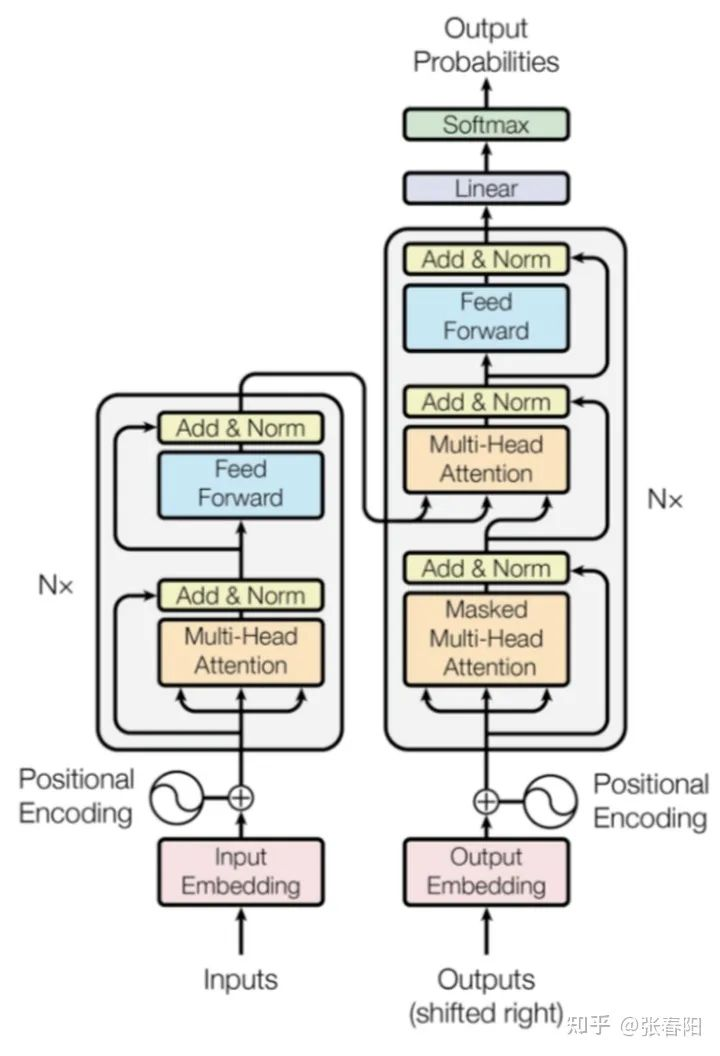class Transformer(nn.Module):        def __init__(self, src_vocab, trg_vocab, d_model, N, heads, dropout):        super().__init__()        self.encoder = Encoder(src_vocab, d_model, N, heads, dropout)        self.decoder = Decoder(trg_vocab, d_model, N, heads, dropout)        self.out = nn.Linear(d_model, trg_vocab)            def forward(self, src, trg, src_mask, trg_mask):        e_outputs = self.encoder(src, src_mask)        d_output = self.decoder(trg, e_outputs, src_mask, trg_mask)        output = self.out(d_output)        return output



https://github.com/BSlience/transformer-all-in-onegithub.com

end03-2821
09-1623009-08134
01-15551
07-205045
03-01191
11-07173
01-031万+
12-196503
01-071万+
03-136666
06-21111
08-2961
04-241518
04-022343
05-12365
05-211032
09-27472
11-241056点击重新获取扫码支付余额充值